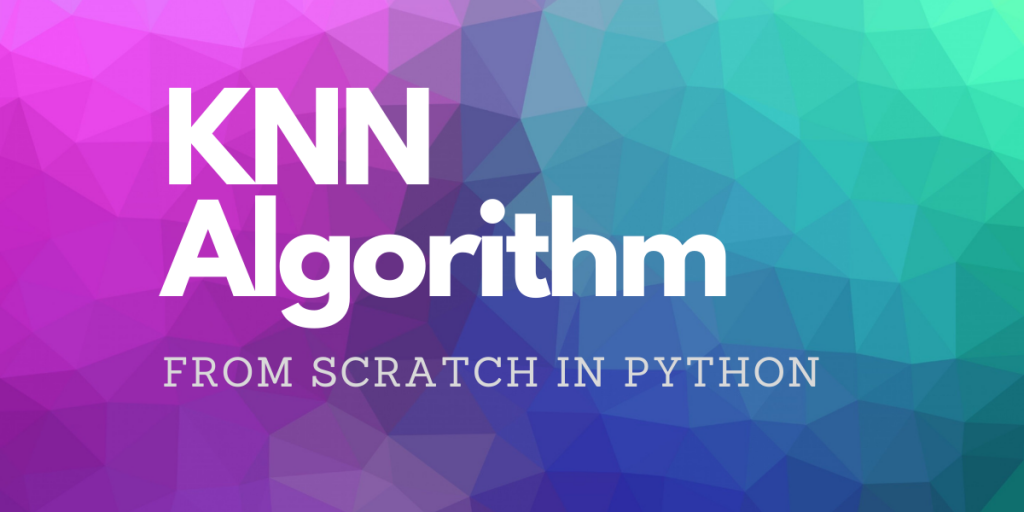# K-Nearest Neighbors from Scratch with PythonIn this article, we’ll learn to implement K-Nearest Neighbors from Scratch in Python. KNN is a Supervised algorithm that can be used for both classification and regression tasks.

KNN is very simple to implement. In this article, we will implement the KNN algorithm from scratch to perform a classification task.

## The intuition behind the K-Nearest Neighbors Algorithm

In K-Nearest Neighbors there is no learning required as the model stores the entire dataset and classifies data points based on the points that are similar to it. It makes predictions based on the training data only.

Consider the figure above. There are two classes of data (Red and Green) and we were given a new data point (black) and asked to specify which class does this new datapoint belongs to?

Well, KNN drives on the notion that similar items tend to be closer in groups. So it is quite evident that the new data point is closer to the red group and hence the algorithm will classify this point as Red. You can read more about the algorithm on its Wiki page

Ways to calculate the distance in KNN:

• Manhattan Method
• Euclidean Method
• Minkowski Method
• mahalanobis distance
• etc..

In this article, we will be using Euclidean distance to calculate the proximity of a new data point from each point in our training dataset.

## Implementing K-Nearest Neighbors from Scratch in Python

First we will figure out the steps involved in the implementation of K-Nearest Neighbors from Scratch.

Step 1. Figure out an appropriate distance metric to calculate the distance between the data points.

Step 2. Store the distance in an array and sort it according to the ascending order of their distances (preserving the index i.e. can use NumPy argsort method).

Step 3. Select the first K elements in the sorted list.

Step 4. Perform the majority Voting and the class with the maximum number of occurrences will be assigned as the new class for the data point to be classified.

## Complete Python code for K-Nearest Neighbors

Now converting the steps mentioned above in code to implement our K-Nearest Neighbors from Scratch

```#Importing the required modules
import numpy as np
from scipy.stats import mode

#Euclidean Distance
def eucledian(p1,p2):
dist = np.sqrt(np.sum((p1-p2)**2))
return dist

#Function to calculate KNN
def predict(x_train, y , x_input, k):
op_labels = []

#Loop through the Datapoints to be classified
for item in x_input:

#Array to store distances
point_dist = []

#Loop through each training Data
for j in range(len(x_train)):
distances = eucledian(np.array(x_train[j,:]) , item)
#Calculating the distance
point_dist.append(distances)
point_dist = np.array(point_dist)

#Sorting the array while preserving the index
#Keeping the first K datapoints
dist = np.argsort(point_dist)[:k]

#Labels of the K datapoints from above
labels = y[dist]

#Majority voting
lab = mode(labels)
lab = lab.mode
op_labels.append(lab)

return op_labels
```

Our predict function requires a Training dataset, True Labels, Datapoints to classify, and the number of nearest neighbor (K) as the input arguments.

### K-Nearest Neighbors from Scratch with the iris dataset

Now it’s time to test our implementation on some data.

```#Importing the required modules
#Importing required modules
from sklearn.metrics import accuracy_score
from numpy.random import randint

# Store features matrix in X
X= iris.data
#Store target vector in
y= iris.target

#Creating the training Data
train_idx = xxx = randint(0,150,100)
X_train = X[train_idx]
y_train = y[train_idx]

#Creating the testing Data
test_idx = xxx = randint(0,150,50) #taking 50 random samples
X_test = X[test_idx]
y_test = y[test_idx]

#Applying our function
y_pred = predict(X_train,y_train,X_test , 7)

#Checking the accuracy
accuracy_score(y_test, y_pred)
```

Output:

```0.98
```

With K equals to 7, our implemented model seems to perform very well on the given data.

## Conclusion

In this article, we implemented our very own K-Nearest Neighbors from Scratch and applied it to a classification problem.

We determined the inner working of the KNN algorithm and looked into the steps involved in making the algorithm. Being so simple KNN is a very powerful and useful algorithm in Machine Learning.

If you’re interested in some related from the scratch implementations, take a look at these articles:

Till we meet next time.

Happy Learning!